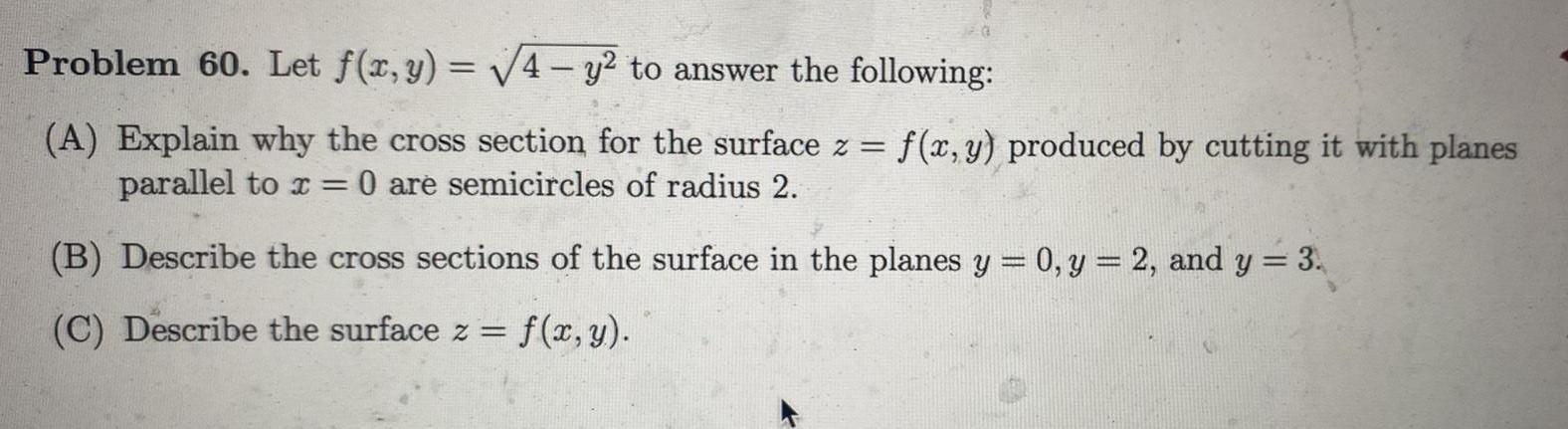Question:

# Problem 60 Let f x y 4 y to answer the following A Explain

Last updated: 7/29/2023Problem 60 Let f x y 4 y to answer the following A Explain why the cross section for the surface z f x y produced by cutting it with planes parallel to x 0 are semicircles of radius 2 B Describe the cross sections of the surface in the planes y 0 y 2 and y 3 C Describe the surface z f x y